Function Repository Resource:

# NumeralRepresentation

Display a representation of an integer in a specified number system

Contributed by: Wolfram|Alpha Math Team
 ResourceFunction["NumeralRepresentation"][n,"system"] displays the integer n in the numeral system specified by the string "system". ResourceFunction["NumeralRepresentation"][n,"system",type] displays the integer n in the numeral system "system", returning output of the specified type.

## Details

Supported numeral systems include "Aegean", "Aztec", "Babylonian", "Cistercian", "Egyptian", "Greek", "Mayan", "Roman" and "Suzhou".
Supported values for type include Interpretation, Graphics and List. Roman numerals can also be returned as a String.
ResourceFunction["NumeralRepresentation"] has the Listable attribute.
ResourceFunction["NumeralRepresentation"] has the same options as Graphics, with the following additions:
 FontColor Black the color of the numerals FontSize Automatic the size of the numerals Spacings 0.5 the spacing between the numerals "NumeralStyle" "Simplified" whether to display the numeral in "Handwritten" or "Simplified" style Tooltip False whether to add tooltips that show the numeral or digit values or names
The option Tooltip can take the values True, False, "PlaceValue", "SymbolValue" or "SymbolName" depending on what should be displayed on mouse hovers.

## Examples

### Basic Examples (2)

Get the representation for 18 in Mayan numerals:

 In:=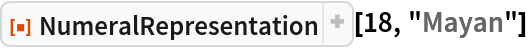Out=Get a list of the numerals that represent 1124640 in Egyptian numerals:

 In:=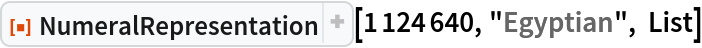Out=### Scope (4)

By default, numerals are returned as an Interpretation of a Graphics object:

 In:=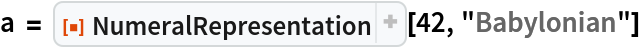Out=In:=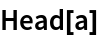Out=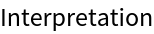Returning numerals with Interpretation allows basic computations with the numerals:

 In:=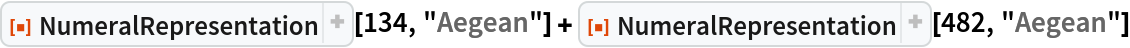Out=In:=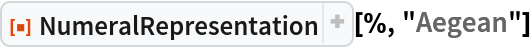Out=The optional third argument also allows you to return numerals as simple Graphics objects or as a list of Graphics objects:

 In:=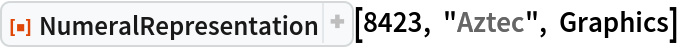Out=In:=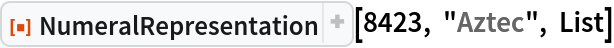Out=For Roman numerals only, String is also a supported output type:

 In:=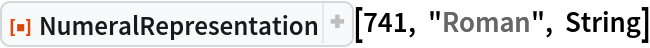Out=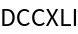#### Aegean (1)

Aegean numerals are a base-10 number system. There are symbols for each power of ten from 1 to 10,000:

 In:=Out=#### Aztec (2)

Aztec numerals are a base-20 system, with additional symbols for 10 and small multiples of 100. There are symbols for the following values: a dot represents 1, a lozenge represents 10, a flag represents 20, feathers with increasing numbers of barbs represent 100, 200, 300 and 400, and a xiquipilli (bag) represents 8000:

 In:=Out=Most integers can be represented in multiple ways. For example, 35 can be 35 dots (35 × 1); three lozenges with five dots (3 × 10 + 5 × 1); or one flag, one lozenge and five dots (1 × 20 + 1 × 10 + 5 × 1). NumeralRepresentation returns just the representation with the fewest number of symbols needed:

 In:=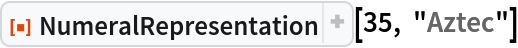Out=#### Babylonian (2)

Babylonian numerals are a base-60 system. There is a symbol for a unit and a symbol for a ten:

 In:=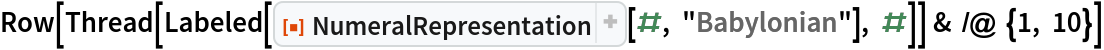Out=Here are the Babylonian numerals for 1 - 60:

 In:=Out=#### Cistercian (2)

Cistercian numerals are a base-10 system where each number from 1 - 9999 has a unique symbol. The symbols have four parts (top right, top left, bottom right and bottom left), each of which corresponds to a place value (ones, tens, hundreds and thousands, respectively):

 In:=Out=Here are the Cistercian numerals for 1 - 9:

 In:=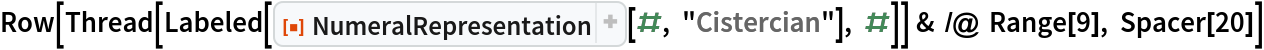Out=#### Egyptian (2)

Egyptian numerals are a base-10 number system. There are symbols for each power of ten from 0 to 6 (i.e., 1 to 1,000,000), each made of a figure representing an object:

 In:=Out=Up to the ten thousands, the Egyptian symbols are arranged in rows or columns to form each place value. For hundred thousands and millions, NumeralRepresentation just places the repeated symbols in a single row:

 In:=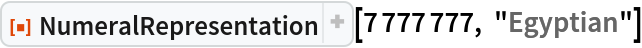Out=#### Greek (2)

Greek numerals are a positional base-10 numeral system. There are separate characters for the digits 1 - 9, the multiples of 10 from 10 - 90, and the multiples of 100 from 100 - 900:

 In:=Out=There is a single character that represents 1000, and multiples of 1000 are distinguished by adding an additional character to its upper left:

 In:=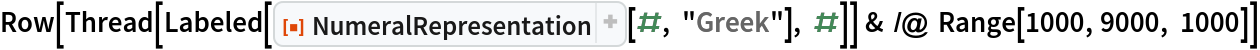Out=#### Mayan (3)

Mayan numerals are a positional base-20 system. There symbols include a dot for one, a bar for five and a shell for zero:

 In:=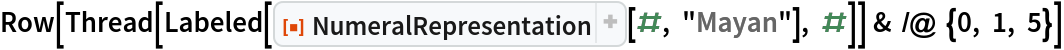Out=Numerals are stacked vertically from bottom to top. The bottom group of symbols represents ones, the next group up represents twenties, the next group up represents four-hundreds (202), and so on. For example, the numeral 1357 is written as follows:

 In:=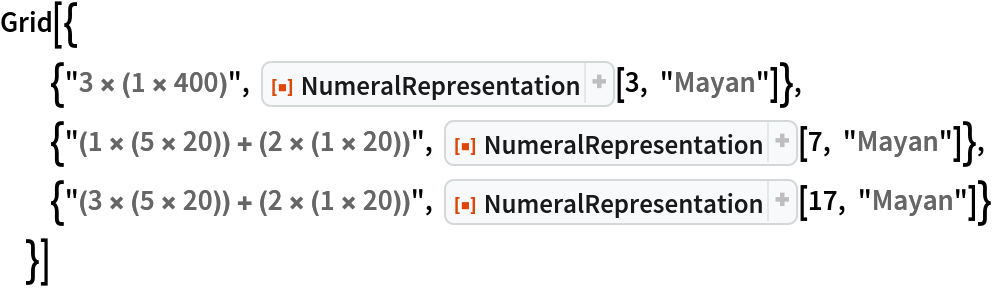Out=Here are the Mayan numerals from 1 to 20:

 In:=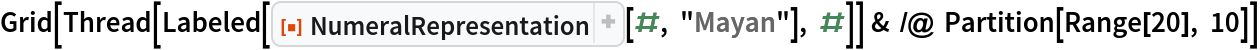Out=#### Roman (2)

Roman numerals are a base-ten number system comprised of seven symbols (1, 5, 10, 50, 100, 500, 1000):

 In:=Out=A vinculum (overbar) represents multiplication by 1000 and can be used to represent large numbers:

 In:=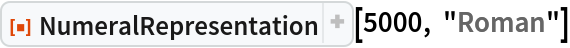Out=#### Suzhou (2)

Suzhou numerals are a positional base-10 system, with separate symbols for the digits 0 - 9. Here are all of the Suzhou numeral symbols:

 In:=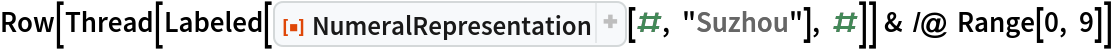Out=When the characters for ones, twos or threes are written consecutively, they are rotated so as not to run together. For example:

 In:=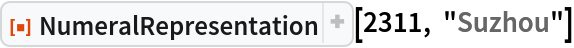Out=### Options (8)

#### NumeralStyle (1)

Each numeral type can be displayed in either a "Simplified" or a "Handwritten" style:

 In:=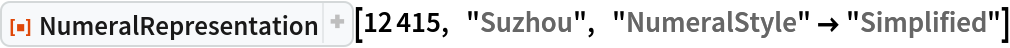Out=In:=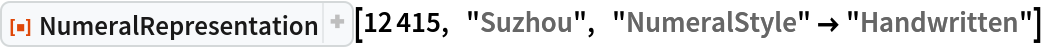Out=In:=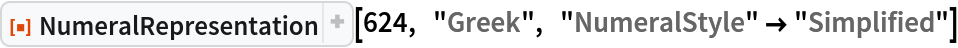Out=In:=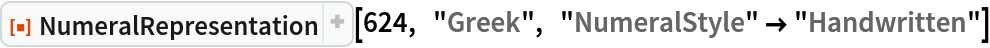Out=#### Tooltip (3)

Setting is the same as setting Tooltip"PlaceValue", where each digit in the numeral has a tooltip displaying its place value:

 In:=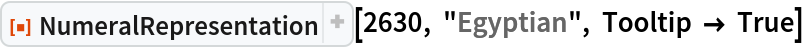Out=Setting Tooltip"SymbolValue" adds a tooltip to each symbol within each digit, showing its value:

 In:=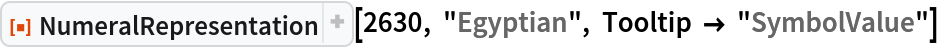Out=Setting Tooltip"SymbolName" adds a tooltip to each symbol within each digit, showing a string describing each symbol:

 In:=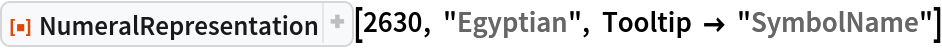Out=#### FontColor (1)

The FontColor option controls the color in which the numerals are rendered:

 In:=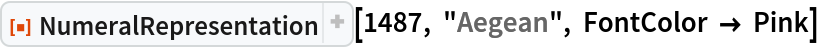Out=#### FontSize (1)

The FontSize option controls the size in which the numerals are rendered:

 In:=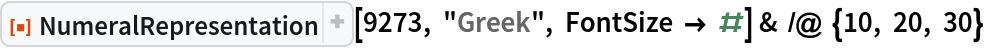Out=#### Spacings (2)

The Spacings option controls the spacing between the numerals:

 In:=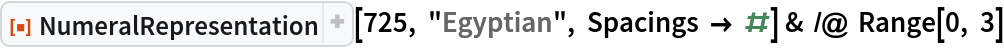Out=For the vertically-arranged Mayan numeral system, Spacings adjusts the vertical spacing of the numerals:

 In:=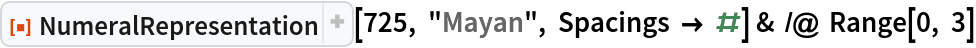Out=### Possible Issues (3)

Several of the numeral systems have an upper limit on the values which have representations:

 In:=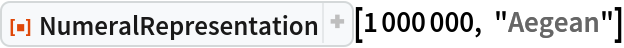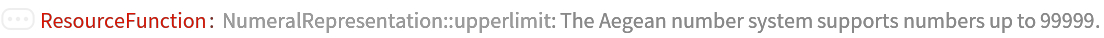Out=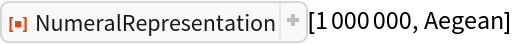Several of the numeral systems have no symbol that represents zero:

 In:=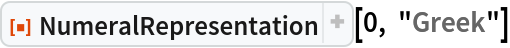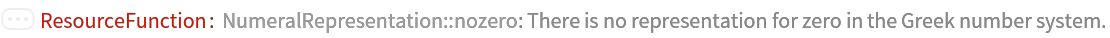Out=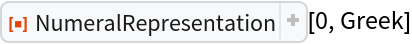Because it is used in the arrangement of the numerals themselves, the PlotRange option of Graphics cannot be given to NumeralRepresentation:

 In:=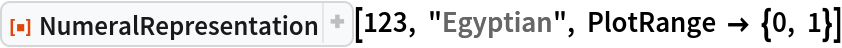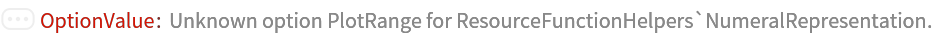Out=## Publisher

Wolfram|Alpha Math Team

## Version History

• 2.0.0 – 23 March 2023
• 1.1.0 – 13 January 2023
• 1.0.0 – 14 October 2022

## Author Notes

To view the full source code for NumeralRepresentation, evaluate the following: